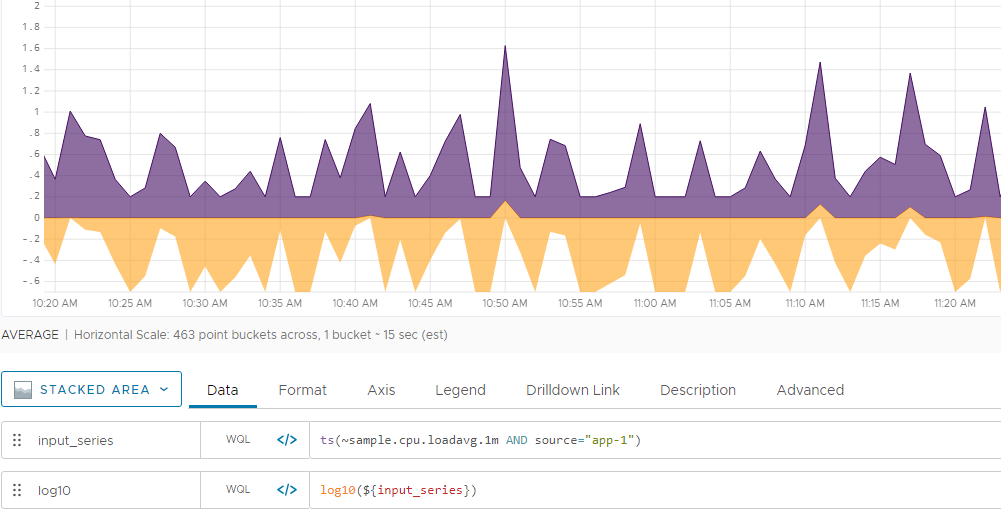Reference to the log10() function

## Summary

``````log10(<tsExpression>)
``````

Returns the logarithm base 10 of each data value described by the expression.

## Parameters

ParameterDescription
tsExpression Expression describing a constant or a time series of values to take the logarithm of.

## Description

The `log10()` function produces a time series in which each data value is equal to the decimal logarithm (log base 10) of the value or values specified by the expression. The decimal logarithm is the power to which you need to raise 10 to obtain the value of the expression.

• If `tsExpression` describes a constant value `N`, then `log10()` returns a continuous series where every data value is `log10 N`.
• If `tsExpression` describes one or more time series, then `log10()` returns a new time series for each input time series. Each value in a new time series is calculated as `log10 V`, where `V` is the value of the corresponding point in the input time series.

By default, the chart legend displays a rounded version of the result. You can cause the legend to display the result with more precision by holding down the shift key when you hover over the time series.

## Examples

Here we see the result (in orange) of using `log10()` to take the decimal log of a time series (in purple).